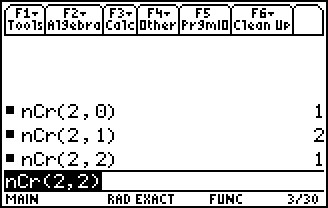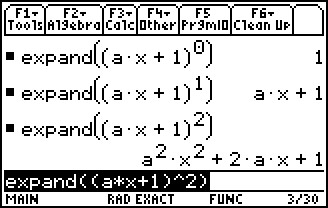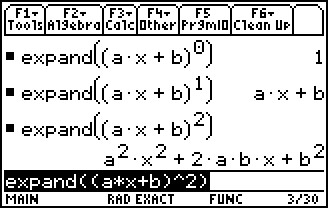# Activities

••• ##### Subject Area

• Math: Precalculus: Sequences
• Math: Precalculus: Series

• ##### Author9-12

45 Minutes

• ##### Device
• TI-83 Plus Family
• TI-84 Plus
• TI-84 Plus Silver Edition
• TI-89 / TI-89 Titanium

## Expanding Binomials#### Activity Overview

In this activity, students will explore Pascal's Triangle and the Binomial Theorem. They will compare the numbers in the triangle to combinations.

#### Key Steps

•In this activity, students will explore Pascal’s Triangle and the Binomial Theorem. They will compare the numbers in the triangle to combinations.

Note: This activity is best utilized with the TI-89 Titanium.

•First, they will expand binomials to investigate the effect of b in the binomial (x + b)n and then see the effect of a in the binomial (ax + 1)n.

•They will then develop a pattern for both variables together in the binomial (ax + b)n in order to state the Binomial Theorem. There are extra problems on the worksheet for students to complete using the theorem.